﻿ Modelling and Forecasting Seasonal Behavior of Rainfall in Abuja, Nigeria; A SARIMA ApproachAmerican Journal of Mathematics and Statistics

p-ISSN: 2162-948X    e-ISSN: 2162-8475

2020;  10(1): 10-19

doi:10.5923/j.ajms.20201001.02### Modelling and Forecasting Seasonal Behavior of Rainfall in Abuja, Nigeria; A SARIMA Approach

1Department of Statistics, University of Abuja, Abuja, Nigeria

Correspondence to: Samuel Olorunfemi Adams, Department of Statistics, University of Abuja, Abuja, Nigeria.
 Email: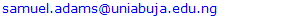Abstract

The need to have a quantitative means of modelling and predicting rainfall is very important for the purposes of Airplane movements, Agriculture, planning and policy formulation. The aim of this study is to propose a model of Seasonal Autoregressive Integrated Moving Average (SARIMA) model to model and forecast the monthly frequency of the rainfall in Abuja, Nigeria for the period 1996 to 2018. The data was obtained from Nigerian Meteorological Agency (NiMet) Abuja, Nigeria and the analysis was based on probability Seasonal time series modelling approach. The Plot of the original data shows that the time series is stationary and the Augmented Dickey-Fuller test did not suggest otherwise. The graph further displays evidence of seasonality and it was removed by seasonal differencing. The plots of the ACF and PACF show spikes at seasonal lags, the minimum Akaike information criterion (AIC) was 3618.5, Bayesian Information Criterion (BIC) was 3624.3, maximum Coefficient of Determination (R2) was 0.799 and all the parameters of the proposed SARIMA model were statistically significant at p < 0.05, suggesting SARIMA (0, 0, 2)(0, 1, 2)12 as the best for modeling and predicting the monthly rainfall in Abuja, Nigeria. The fitted model was used to make forecast for the next four proceeding years, the average predicted rainfall for the peak periods i.e. April, May, June, July, August and September for the years; 2019, 2020, 2021 and 2022 were; 335.5mm, 337.5mm, 339.3mm and 341.2mm respectively, this implies; a 3.5%, increase in rainfall from 2018 to 2019, 4.% increase in rainfall from 2018 to 2020, 4.7%, increase in rainfall from 2018 to 2021 and a 5.2%, increase in rainfall from 2018 to 2022. The results are useful for predicting the expected rainfall in Abuja in the next four years and also provide information that would be helpful for decision makers in formulating policies, planning and mitigating the problems of flooding in Abuja. Thus, the study recommends among others that Federal Government and minister of the federal capital territory, Abuja should commence the process of avoiding flood by building dikes, levees and provision of adequate drainage systems in the city.

Keywords: ACF, Seasonality, Stationarity, PACF, Rainfall, SARIMA

Cite this paper: Samuel Olorunfemi Adams, Muhammad Ardo Bamanga, Modelling and Forecasting Seasonal Behavior of Rainfall in Abuja, Nigeria; A SARIMA Approach, American Journal of Mathematics and Statistics, Vol. 10 No. 1, 2020, pp. 10-19. doi: 10.5923/j.ajms.20201001.02.

### 1. Introduction

Rainfall is the total amount of separate water drops which fall to the earth from the clouds, having been formed by the condensation of water vapor in the atmosphere. Condensation of this water vapor is brought about by the rise of air considerable height above the earth’s surface, during a given time, rainfall is measure by an instrument call rain gauge.  defined rainfall intensity duration frequency relationships as graphical representations of the amount of water that falls within a given period of time. These graphs are used to determine when an area will be flooded, and when a certain rainfall rate or a specific volume of flow will reoccur in the future. According to , rainfall frequency analyses are used extensively in the design of systems to handle storm runoff, including roads, culverts and drainage systems.  States that “the precipitation frequency analysis problem is to compute the amount of precipitation y falling over a given area in a duration of x minutes with a given probability of occurrence in any given year.” For engineering design applications, it is necessary to specify the temporal distribution of rainfall for a given frequency, or return interval. According to , IDF or Depth-Duration-Frequency (DDF) curves “allow calculation of the average design rainfall intensity [or depth] for a given expedience probability over a range of durations” and is the result of the rainfall frequency analysis. IDF estimates are important statistical summaries of precipitation records used for hydrologic engineering design .
The rain in Nigeria is characterized by high intensity rainfall, high frequency of rain occurrence and the increased presence of large raindrops when compared with temperate climates . In Southeastern Nigeria, IDF curves are not readily available. Also the number of years of data used to develop a few IDF curves for the region found in the literature were rather short,  and . A lot of attention has been directed towards modelling and forecasting the amount of rainfall in various parts of Nigeria. For example: In Ikeja, Lagos state , , Sahel Region in Nigeria, , Bauchi, , climatic zones in Nigeria, , Akure, Ondo state , Osun State , , Ile-Ife, Osun State , Umuahia, Abia State , Ota, Ogun State , Ogbomosho, Oyo State , ilorin .
There are also vast studies on modelling and forecasting of the frequency of rainfall outside Nigeria. In Bangladesh , Iran , Jordan , Golastan Province , East Africa  and Turkey .
The aim of this study is to propose a Seasonal Autoregressive Integrated Moving Average (SARIMA) model for modelling and forecasting the monthly rainfall in Abuja for the period 1996 – 2018. Following the introduction, theoretical framework is presented in section 2, review of related literatures in section 3. Section 4 presents the materials and methods of the study. Section 5 presents results and discussions and finally, conclusions and recommendations are presented in section 6.

### 2. Theoretical Framework

#### 2.1. Study Area

Abuja is the capital city of Nigeria located in the centre of the country within the Federal Capital Territory (FCT). It is a planned city and was built mainly in the 1980s, replacing the country's most populous city of Lagos as the capital on 12th December 1991.  Abuja's geography is defined by Aso Rock, a 400-metre (1,300 ft) monolith left by water erosion. The Presidential Complex, National Assembly, Supreme Court and much of the city extend to the south of the rock. Zuma Rock, a 792-metre (2,598 ft) monolith, lies north of the city on the expressway to Kaduna. At the 2006 census, the city of Abuja had a population of 776,298,  making it one of the ten most populous cities in Nigeria. According to the United Nations, Abuja grew by 139.7% between 2000 and 2010, making it the fastest growing city in the world, . As of 2015, the city is experiencing an annual growth of at least 35%, retaining its position as the fastest-growing city on the African continent and one of the fastest-growing in the world, . As at 2016, the metropolitan area of Abuja is estimated at eight million persons, placing it behind only Lagos, as the most populous metro area in Nigeria, .
Abuja experiences three weather conditions annually, this includes a warm, humid rainy season and an extremely hot dry season. In between these seasons, there is a short period of harmattan accompanied by the North East Trade Wind, with the main feature of dust haze, intensified coldness and dryness. The rainy season begins from April and ends in October, when daytime temperatures reach 28-30 degrees and night time lows range around 22-23 degrees. In the dry season, daytime temperatures can soar to 40 degrees and night time temperatures can drop to 12 degrees, resulting in chilly evenings. Even the chilliest nights can be followed by daytime temperatures well above 30 degrees. The high altitudes and rolling terrain of the FCT act as moderating influence on the weather of the territory. Rainfall in the FCT reflects the territory's location on the windward side of the Jos Plateau and the zone of rising air masses. The annual total rainfall is in the region of 1100 mm to 1600 mm.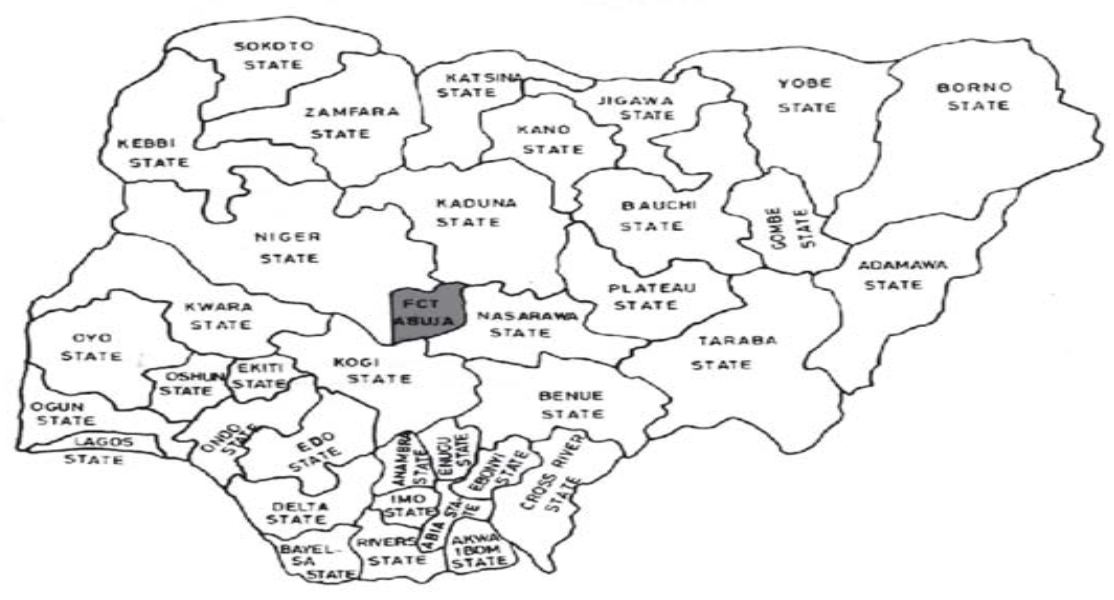Figure 1. Map of Nigeria showing Abuja, Federal Capital Territory

### 4. Material and Methods

#### 4.1. Data Source

The data used in this study is a secondary data of the monthly rainfall (mm), it was obtained from Nigeria Meteorological Agency (NiMet), Abuja station, Nigeria. The data spanned between the periods 1996 to 2018 (twenty-two years).

#### 4.2. Seasonal Autoregressive Integrated Moving Average (SARIMA) Model

Seasonal Autoregressive Integrated Moving Average (SARIMA) model is an algebraic statement that describes how a time series is statistically related to its own past. The seasonal ARIMA model incorporates both non-seasonal and seasonal factors in a multiplicative model given as;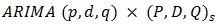(1)
Where; p = non-seasonal AR order, d = non-seasonal differencing, q = non-seasonal MA order, P = seasonal AR order, D = seasonal differencing, Q = seasonal MA order, and S = time span of repeating seasonal pattern.
Without differencing operations, the model could be written more formally as;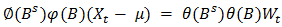(2)
The non-seasonal components are: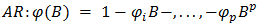(3)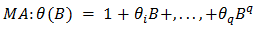(4)
The seasonal components are: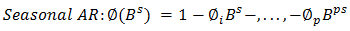(5)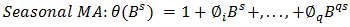(6)
Note that on the left side of equation (2) the seasonal and non-seasonal AR components multiply each other, and on the right side of equation (2) the seasonal and non-seasonal MA components multiply each other (5).
The SARIMA modeling approach is concerned with finding a parsimonious seasonal ARIMA model that describes the underlying generating processed of the observed time series. Box and Jenkins  established a three step modeling procedure: identification, estimation and diagnostic checking steps. The identification step is to tentatively choose one or more ARIMA/SARIMA model(s) using the estimated ACF and PACF plots. The ACF plot of the AR (Auto Regressive)/ SAR (Seasonal Auto Regressive) process shows an exponential decay while its PACF plot truncates at lag p seasonal lag p and diminishes to zero afterwards. The ACF plot of the MA process truncates to zero after lag q while its PACF decays exponentially to zero. The two processes: AR (p)/SAR(P) and MA (q)/SMA(Q), could be combined to form the ARMA (p, q)/SARMA (P, Q) process which has ACF and PACF that decays exponentially to zero. The maximum likelihood estimation method could be used to estimate the parameters of the identified model(s) in the identification stage. The last diagnostic checking stage involves assessing the adequacy of the identified and fitted models through possible statistically significant test on the residuals to verify its consistency with the white noise process e.g. the Ljung-Box test . Finally, the best fitting model would be selected among other satisfactory, competing models e.g. the information criteria statistics on the basis of the AIC or BIC  rule of thumb (Models with the smallest information criterion is the best) and forecast is made with the model of best fit.
For multiplicative Seasonal Autoregressive Integrated Moving Average (SARIMA) model, we have the general notation (p, d, q), (P, D, Q) S where (p, d, q) is the non-seasonal part and (P, D, Q) S is the seasonal part with p, d, q having their usual meaning and P is the order of the seasonal AR process. D is the differencing of the seasonal process, Q is the order of seasonal MA process of the time series, and S is the order of seasonality. For the purpose of identifying a seasonal ARMA process, we divide the process into two parts. To identify the seasonal pattern, we ignore the non-seasonal process and determine whether the seasonality is determined by an AR or an MA process by focusing on the coefficients of the seasonal terms. Suppose that the non-seasonal part is an ARIMA (1, 0, 1) and the time series shows a yearly seasonal pattern, then the complete model becomes;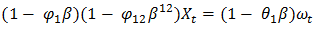, if seasonality is on the AR portion, and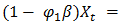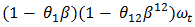, if seasonality is on the MA portion, where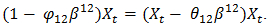Identification of the ARIMA model: Three goodness-of fit statistics that are most commonly used for the model selection are; Coefficient of determination (R2), Akaike Information Criterion (AIC) and Schwarz Bayesian Information Criterion (BIC) [41-42]. The AIC and BIC are determined based on a likelihood function. The AIC and BIC are calculated using the formulas below: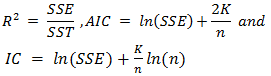(7)
Where; n is the total number of observations, SST is the sum of the squared total, SSE is the sum of the squared errors, and### 5. Results and Discussion

From the plots in Figure 2 it could be seen that the time series plot displays a wave like pattern an evidence of seasonality and no trend is observed which implies that the time series is stationary. The sinusoidal or periodic pattern in the ACF plot is again suggesting that the series has a strong seasonal effect also, the PACF plot is neither suggesting otherwise. In order to verify the stationarity claim of the visual displays an Augmented Dickey-Fuller test  was performed with hypothesis;
H0: The rainfall data is unit root non stationary
and
H1: The rainfall data is stationary.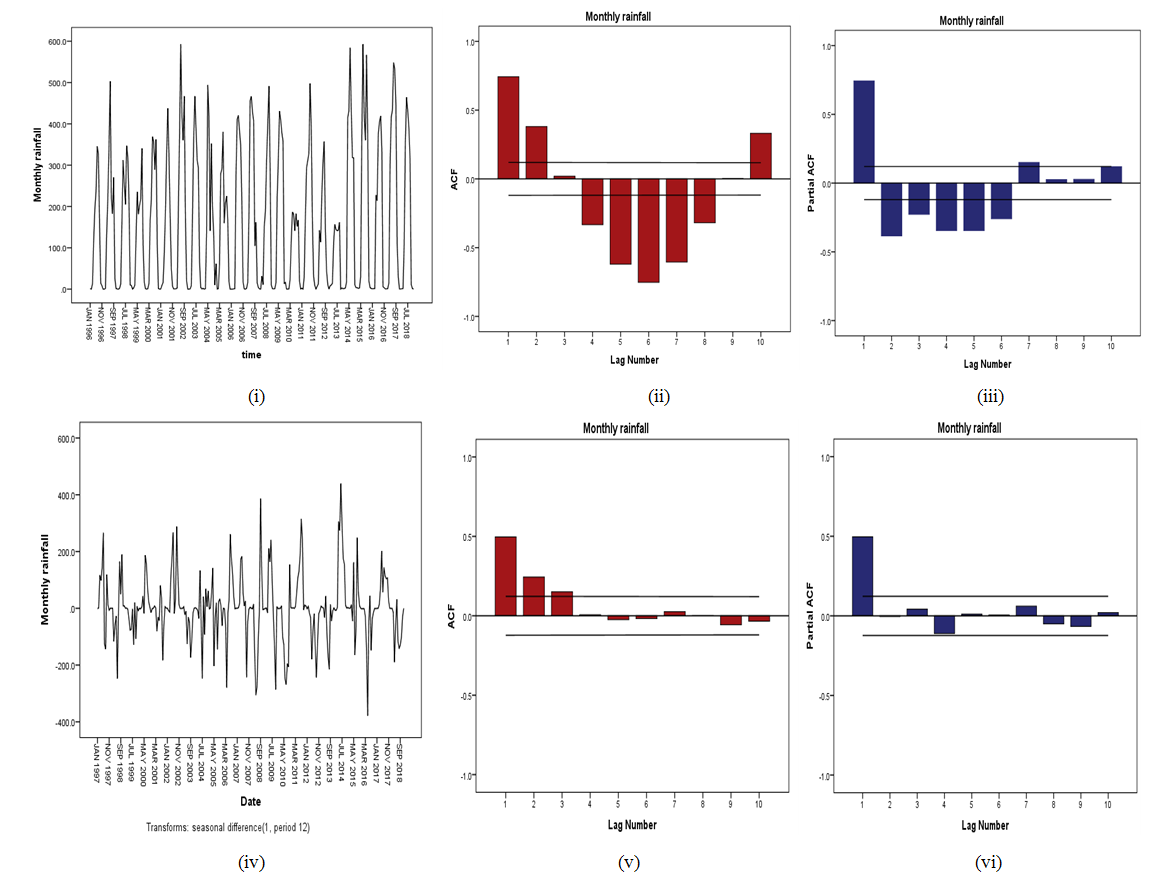Figure 2. (i) Time Series Plot of the Original data, (ii) & (iii)-ACF and PACF Plots Respectively of the Original data, (iv) Time Series Plot of the seasonal Differenced s, (v) & (vi)-ACF and PACF Plots Respectively of the Differenced Logged-data
Decision: Since p-value = 0.01 is less than level of significant = 0.05, the alternative hypothesis is accepted. Thus, strong evidence against the null hypothesis at 5% level of significance. In order to eliminate the seasonal effect from the time series the data was subject to a seasonal differencing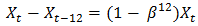and the data is re-examined visually. All the plots in Figure 3 shows that the first seasonally differenced rain fall series in now well behaved.
The ACF of the original un-difference series show a significant positive cut-off at lag 1, 2 and 10, suggesting MA (q) = 1, 2,10, while the PACF of the original non-difference series show a significant positive cut-off at lag 1, suggesting AR (p) = 1. The pronounced spikes at the first, second and third seasonal lags of the ACF suggest, MA (Q) = 1,2,3 and the spike at the first seasonal lag of PACF plot suggests, AR (P) =1 with first seasonal difference (D) = 1, Therefore, proposed Seasonal ARIMA are; SARIMA (0, 0, 1)(1, 1, 1)12, SARIMA (0, 0, 2)(0, 1, 2)12 and SARIMA (0, 0,10)(0, 1, 3)12. The suggested SARIMA models were compared based on the following criteria; coefficient of the proposed models, t-statistics, p-values, standard errors, R2, AIC and BIC respectively. The SARIMA (0, 0, 2)(0, 1, 2)12 clearly proved to be the appropriate model with minimum Akaike information criterion (AIC) of 3618.5, Bayesian Information Criterion (BIC) of 3624.3 and maximum Coefficient of Determination (R2) of 0.799 and all the parameters of the fitted SARIMA (0, 0, 2)(0,1,2) model in Table 2 are statistically significant at p < 0.05. The diagnostic check of the fitted SARIMA (0, 0, 2)(0, 1, 2) model is presented in Figure 3(i-ii), it shows the overall adequacy of the fitted model, where the standardized residual plot shows that the residuals are standard normal distributed on N (0, 1), the ACF and PACF plot also shows that the residuals are uncorrelated at the lags. Fig. 3 shows the time series of the standardized residuals. The plot depicts no pattern, a clear indication that the standardized residuals behaves like a typical white noise process as required. The goodness of fit of the two tentatively identified models would be judged on the basis of their information criterion statistics (BIC) and Akaike Information Criterion (AIC) .
 Table 1. Augmented Dickey Fuller Test of Abuja monthly rainfall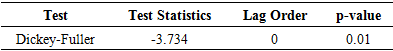Table 2. Suggested SARIMA Models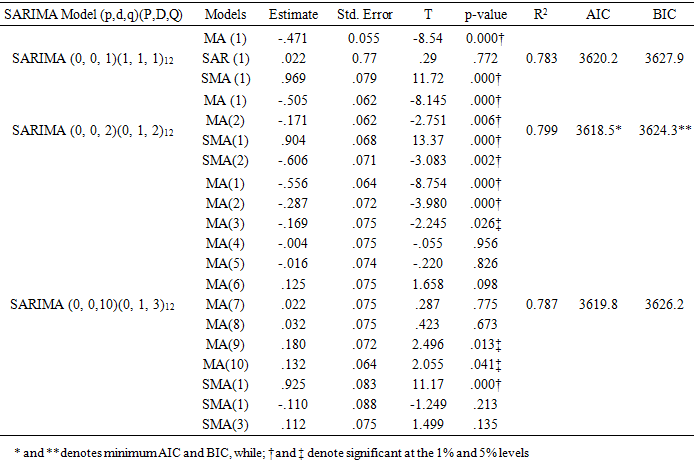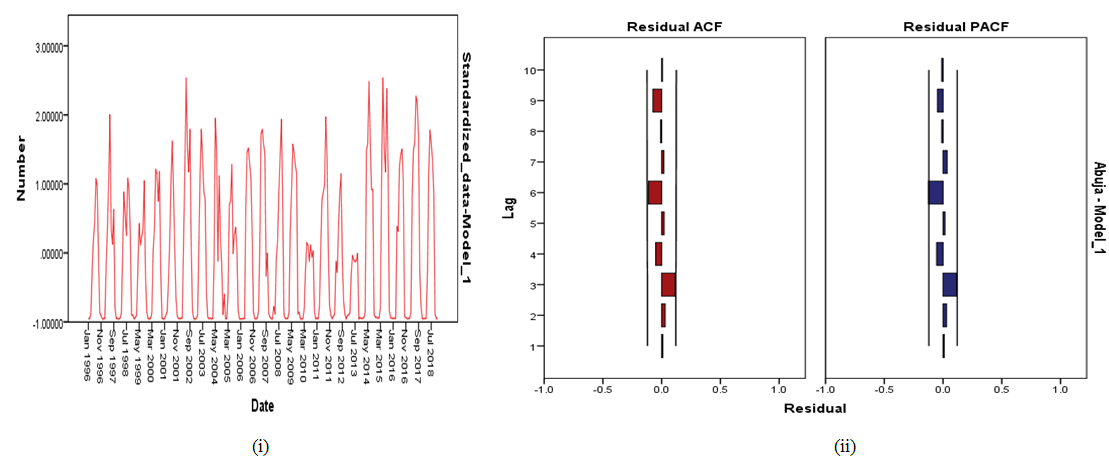Figure 3. (i) The Standardized Residual Plot of the Fitted SARIMA(0,0,2)(0, 1, 2) and (ii) ACF and PACF Plots Respectively of the standardized residual of the fitted SARIMA (0,0,2)(0,1,2) Model

#### 5.1. Forecasting with the Fitted Models

The selected model was then used to describe and forecast the time series observations for the next three years (January 2019 – December 2022) as presented in table 3. Using SARIMA (0, 0, 2)(0, 1, 2) Model, the average monthly rainfall in 2018 was 173.4mm, while the average predicted monthly rainfall for year 2019, 2020, 2021 and 2022 were; 184.8mm, 186.9mm, 188.8mm and 190.7mm respectively, this implies that, there will be a 6.6%, increase in rainfall from 2018 to 2019, 7.8% increase in rainfall from 2018 to 2020, 8.9%, increase in rainfall from 2018 to 2021 and a 10.0%, increase in rainfall from 2018 to 2022. Fig. (4) Shows the past (red lines) and forecast (blue lines) time series of the original monthly rainfall data in Abuja, with the fitted SARIMA model while the dashed lines in both plots indicate the 95% confidence intervals.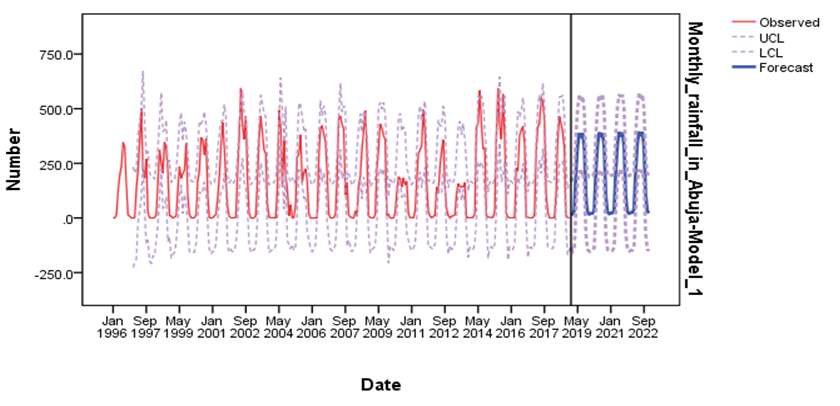Figure 4. Forecast of the Monthly rainfall in Abuja and its 95% confidence interval
 Table 3. Forecasts from period January 2019 to December 2022 (95 Percent Confidence Interval)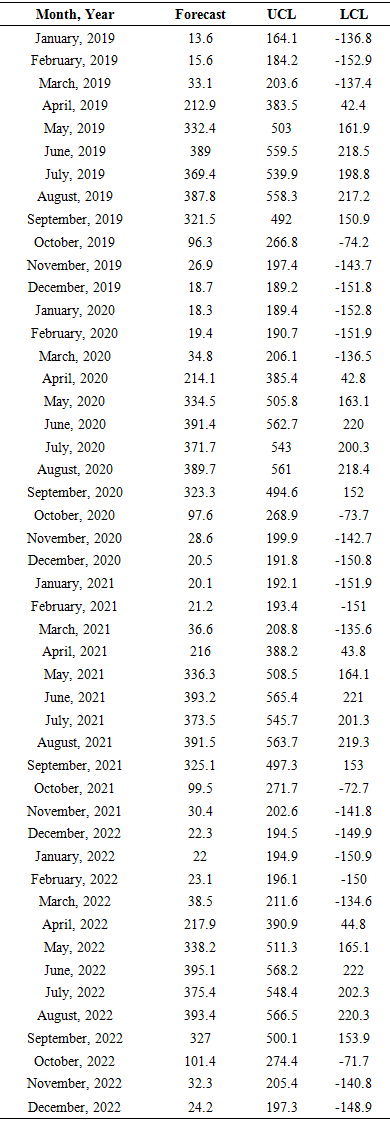To validate or test how well the fitted SARIMA (0, 0, 2) (0, 1, 2)12 model has performed in forecasting the future frequency of monthly rainfall in Abuja, the observed and forecast values of the model were subjected to a two sample Student's t-test as shown below.
Hypothesis:Vs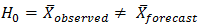Table 4. T-test result of the observed and forecast value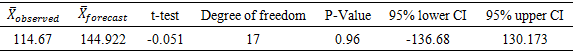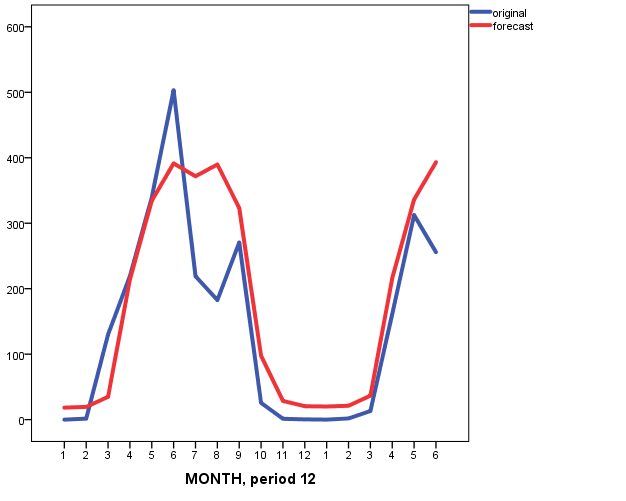Figure 5. Time series of monthly rainfall forecast( red line) and observed monthly (blue line) rainfall in Abuja
The result presented in table 4 and figure 5 above indicates that there is no statistically significant difference between the observed and forecast frequency of monthly rainfall values in Abuja at 5% level of significance. This is because the p-value = 0.960 is greater than 0.05. Alternatively, it could equally be inferred from the 95% confidence interval [-136.68, 130.173] because the interval contains zero. Thus, the SARIMA (0, 0, 2) (0, 1, 2)12 model has forecast the frequency of monthly rainfall in Abuja efficiently.

### 6. Conclusions and Recommendations

In this study, the frequency of monthly rainfall from 1996 to 2018 obtained from Nigeria Meteorological Agency (NiMet), Abuja station, Nigeria. The data spanned between the period 1996 to 2018 (twenty-two years). The data was analysed using probability time series modelling approach. The Plot of the original data shows that the time series is stationary and has evidence of seasonality. The Augmented Dickey-Fuller test confirmed the stationarity claim. Seasonal differencing was done to remove the seasonal effect. SARIMA modelling of the data was upheld after duly following the conventional three steps of identification, estimation and diagnostic checking procedures established by Box and Jenkins. The SARIMA (0, 0, 2)(0, 1, 2)12 clearly proved to be the appropriate model with minimum Akaike information criterion (AIC) of 3618.5, Bayesian Information Criterion (BIC) of 3624.3 and maximum Coefficient of Determination (R2) of 0.799 and all the parameters of the fitted SARIMA (0, 0, 2)(0,1,2) model were statistically significant was used to make forecast. The average rainfall in the four months peak periods in 2018 was 324.2mm, while the average predicted rainfall for the peak periods i.e. April, May, June, July, August and September for the years; 2019, 2020, 2021 and 2022 were; 335.5mm, 337.5mm, 339.3mm and 341.2mm respectively, this implies that, there will be a 3.5%, increase in rainfall from 2018 to 2019, 4.1% increase in rainfall from 2018 to 2020, 4.7%, increase in rainfall from 2018 to 2021 and a 5.2%, increase in rainfall from 2018 to 2022. Comparison of the observed and forecasted frequency of some selected values analyzed with a t-test showed no significant difference.
The results are useful for predicting the expected rainfall in Abuja in the next four years and also provide information that would be helpful for decision makers in formulating policies to mitigate the problems of flooding in Abuja. The percentage increments in the predicted rainfall frequency in Abuja, Nigeria is an indication of flood, it is therefore recommended that the Federal Government and minister of the federal capital territory, Abuja should commence the process of avoiding flood by building dikes, levees and provision of adequate drainage systems.